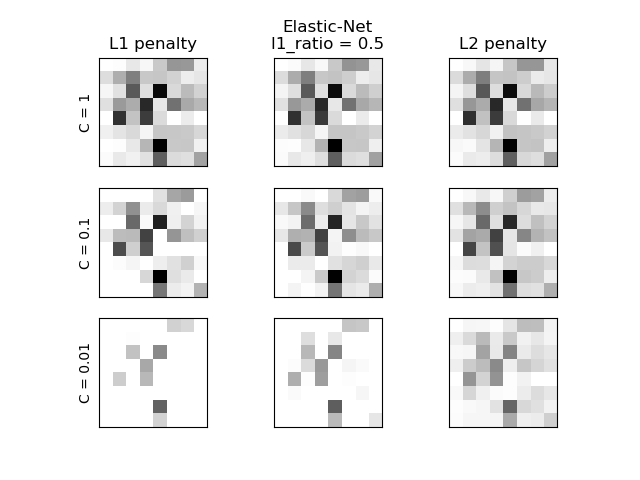# L1 Penalty and Sparsity in Logistic Regression¶

Comparison of the sparsity (percentage of zero coefficients) of solutions when L1, L2 and Elastic-Net penalty are used for different values of C. We can see that large values of C give more freedom to the model. Conversely, smaller values of C constrain the model more. In the L1 penalty case, this leads to sparser solutions. As expected, the Elastic-Net penalty sparsity is between that of L1 and L2.

We classify 8x8 images of digits into two classes: 0-4 against 5-9. The visualization shows coefficients of the models for varying C.Out:

C=1.00
Sparsity with L1 penalty:                6.25%
Sparsity with Elastic-Net penalty:       4.69%
Sparsity with L2 penalty:                4.69%
Score with L1 penalty:                   0.90
Score with Elastic-Net penalty:          0.90
Score with L2 penalty:                   0.90
C=0.10
Sparsity with L1 penalty:                29.69%
Sparsity with Elastic-Net penalty:       12.50%
Sparsity with L2 penalty:                4.69%
Score with L1 penalty:                   0.90
Score with Elastic-Net penalty:          0.90
Score with L2 penalty:                   0.90
C=0.01
Sparsity with L1 penalty:                84.38%
Sparsity with Elastic-Net penalty:       68.75%
Sparsity with L2 penalty:                4.69%
Score with L1 penalty:                   0.86
Score with Elastic-Net penalty:          0.88
Score with L2 penalty:                   0.89


print(__doc__)

# Authors: Alexandre Gramfort <alexandre.gramfort@inria.fr>
#          Mathieu Blondel <mathieu@mblondel.org>
#          Andreas Mueller <amueller@ais.uni-bonn.de>

import numpy as np
import matplotlib.pyplot as plt

from sklearn.linear_model import LogisticRegression
from sklearn import datasets
from sklearn.preprocessing import StandardScaler

X, y = digits.data, digits.target
X = StandardScaler().fit_transform(X)

# classify small against large digits
y = (y > 4).astype(np.int)

l1_ratio = 0.5  # L1 weight in the Elastic-Net regularization

fig, axes = plt.subplots(3, 3)

# Set regularization parameter
for i, (C, axes_row) in enumerate(zip((1, 0.1, 0.01), axes)):
# turn down tolerance for short training time
clf_l1_LR = LogisticRegression(C=C, penalty='l1', tol=0.01, solver='saga')
clf_l2_LR = LogisticRegression(C=C, penalty='l2', tol=0.01, solver='saga')
clf_en_LR = LogisticRegression(C=C, penalty='elasticnet', solver='saga',
l1_ratio=l1_ratio, tol=0.01)
clf_l1_LR.fit(X, y)
clf_l2_LR.fit(X, y)
clf_en_LR.fit(X, y)

coef_l1_LR = clf_l1_LR.coef_.ravel()
coef_l2_LR = clf_l2_LR.coef_.ravel()
coef_en_LR = clf_en_LR.coef_.ravel()

# coef_l1_LR contains zeros due to the
# L1 sparsity inducing norm

sparsity_l1_LR = np.mean(coef_l1_LR == 0) * 100
sparsity_l2_LR = np.mean(coef_l2_LR == 0) * 100
sparsity_en_LR = np.mean(coef_en_LR == 0) * 100

print("C=%.2f" % C)
print("{:<40} {:.2f}%".format("Sparsity with L1 penalty:", sparsity_l1_LR))
print("{:<40} {:.2f}%".format("Sparsity with Elastic-Net penalty:",
sparsity_en_LR))
print("{:<40} {:.2f}%".format("Sparsity with L2 penalty:", sparsity_l2_LR))
print("{:<40} {:.2f}".format("Score with L1 penalty:",
clf_l1_LR.score(X, y)))
print("{:<40} {:.2f}".format("Score with Elastic-Net penalty:",
clf_en_LR.score(X, y)))
print("{:<40} {:.2f}".format("Score with L2 penalty:",
clf_l2_LR.score(X, y)))

if i == 0:
axes_row.set_title("L1 penalty")
axes_row.set_title("Elastic-Net\nl1_ratio = %s" % l1_ratio)
axes_row.set_title("L2 penalty")

for ax, coefs in zip(axes_row, [coef_l1_LR, coef_en_LR, coef_l2_LR]):
ax.imshow(np.abs(coefs.reshape(8, 8)), interpolation='nearest',
cmap='binary', vmax=1, vmin=0)
ax.set_xticks(())
ax.set_yticks(())

axes_row.set_ylabel('C = %s' % C)

plt.show()


Total running time of the script: ( 0 minutes 0.659 seconds)

Gallery generated by Sphinx-Gallery